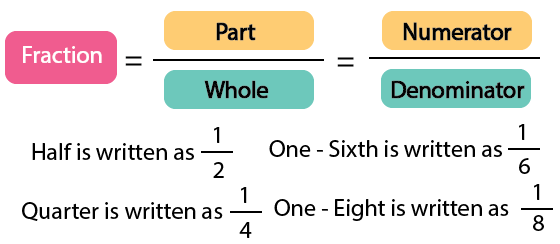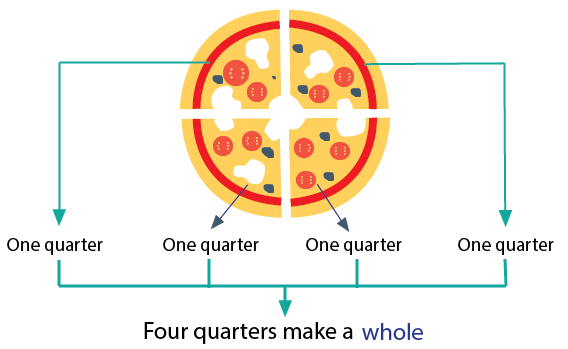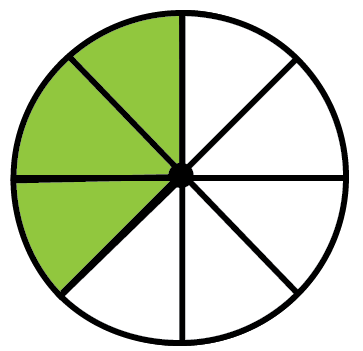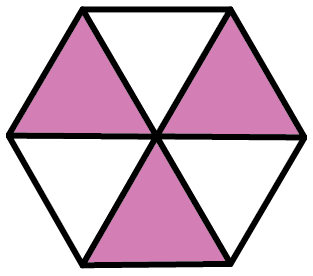Courses
Courses for Kids
Free study material
Free LIVE classes
More

# Parts and Wholes Class 5 Notes CBSE Maths Chapter 4 (Free PDF Download)LIVE
Join Vedantu’s FREE Mastercalss

## NCERT Class 5 Maths Chapter 4: Parts and Wholes Revision Notes and Worksheets

Download NCERT Revision Notes Class 5 Chapter 4 for free from Vedantu. You must have learned what halves and quarters are in the previous classes. In this chapter, you will study what fractions are in Chapter 4 Parts and Wholes. Here, you will study fractions in a unique way. The story-based arithmetic questions will teach how to determine fractions. To understand the fundamental concepts of fractions refer to the Parts and Wholes revision notes available on Vedantu.

The subject experts have compiled these notes to explain the concepts of this chapter in a simple way. You can go through the Parts and Wholes summary to understand the objective of this chapter perfectly.

Last updated date: 23rd Sep 2023
Total views: 143.4k
Views today: 1.43k

## Revision Notes Class 5 Mathematics Chapter 4 - Parts and Wholes

### What is a Fraction?

• Fraction is a part of a whole or a part of a collection.

• Fraction has two parts.

(i) Numerator

(ii) DenominatorFraction Formula

• 4 one-fourth make a whole.Combination of quarters

Solved Example: Find the fraction of the coloured part in the shape.Representation of fraction

1. $\frac{4}{8}$

1. $\frac{5}{8}$

2. $\frac{3}{8}$

3. $\frac{6}{8}$

Ans: There is a total of 8 parts so the denominator of fraction = 8.

We want to find the fraction of the coloured parts.

There is a total of 3 coloured parts so the numerator of fraction = 3.

So the required fraction = $\frac{3}{8}$.

Therefore, Option (C) is correct.

### Fraction of a Group

• The fraction of a group is written as fraction x Group.

Solved Example 1: Find the value of one-Sixth of 48.

Ans: One-Sixth = $\frac{1}{6}$

The value of one-Sixth of 48 = $\frac{1}{6}\times 48=8$

### Types of Fractions

1. Like Fractions

Fractions which have the same denominators are known as Like fractions.

Solved Example: Which of the following pairs of fractions are like fractions?

1. $\frac{4}{8}$ and $\frac{5}{7}$

1. $\frac{5}{7}$ and $\frac{5}{6}$

2. $\frac{3}{5}$ and $\frac{2}{5}$

3. $\frac{6}{8}$ and $\frac{5}{7}$

Ans: Fractions which have the same denominators are known as Like fractions.

• For option A, ($\frac{4}{8}$ and $\frac{5}{7}$) denominators are not the same so these are not like fractions.

• For option B, ($\frac{5}{7}$ and $\frac{5}{6}$) denominators are not the same so these are not like fractions.

• For option C, ($\frac{3}{5}$ and $\frac{2}{5}$) denominators are the same so these are like fractions.

• For option D, ($\frac{6}{8}$ and $\frac{5}{7}$ ) denominators are not the same so these are not like fractions.

Therefore, Option (C) is correct.

2. Unlike Fractions

Fractions which have different denominators are known as the Unlike fractions.

Solved Example: Which of the following pairs of fractions are unlike fractions?

1. $\frac{4}{8}$ and $\frac{5}{8}$

1. $\frac{5}{7}$ and $\frac{6}{7}$

2. $\frac{3}{5}$ and $\frac{2}{5}$

3. $\frac{6}{8}$ and $\frac{5}{7}$

Ans: Fractions which have different denominators are known as the Unlike fractions.

• For option A, ($\frac{4}{8}$ and $\frac{5}{8}$) denominators are the same so these are not unlike fractions.

• For option B, ($\frac{5}{7}$ and $\frac{6}{7}$) denominators are the same so these are not unlike fractions.

• For option C, ($\frac{3}{5}$ and $\frac{2}{5}$) denominators are the same so these are unlike fractions.

• For option D, ($\frac{6}{8}$ and $\frac{5}{7}$) denominators are not the same so these are unlike fractions.

Therefore, Option (D) is correct.

3. Proper Fractions

• Fractions having numerators smaller than the denominator are known as Proper fractions.

• Proper Fractions have value less than 1.

Solved Example: Which of the following fractions is a proper fraction?

1. $\frac{9}{8}$

2. $\frac{10}{8}$

3. $\frac{11}{8}$

4. $\frac{6}{8}$

Ans: Fractions having numerators smaller than the denominator are known as Proper fractions.

In option D, the numerator is 6 and the denominator is 8. So the numerator is less than the denominator.

Therefore $\frac{6}{8}$ is a proper fraction.

Therefore, Option (D) is correct.

4. Improper Fractions

• Fractions having numerators greater than the denominator are known as Improper fractions.

• Improper Fractions have a value as 1 or greater than 1.

Solved Example: Which of the following fractions is an improper fraction?

1. $\frac{5}{8}$

2. $\frac{10}{8}$

3. $\frac{4}{8}$

4. $\frac{6}{8}$

Ans: Fractions having numerators greater than the denominator are known as improper fractions.

In option B, the numerator is 10 and the denominator is 8. So the numerator is greater than the denominator.

Therefore $\frac{10}{8}$ is an improper fraction.

Therefore, Option (B) is correct.

5. Mixed Fractions

• The combination of a whole number and a fraction is known as a Mixed fraction.

Solved Example: Convert $\frac{5}{3}$ into Mixed fractions.

Ans: The combination of a whole number and a fraction is known as a Mixed fraction.

We will divide the numerator by the denominator and the quotient will be the whole number and the remainder will be the numerator of the fraction of mixed fraction and the denominator will be the same.

If we divide 5 by 3 then the quotient will be 1 and the remainder will be 2.
$\frac{5}{3}$ =1$\frac{2}{3}$

Equivalent Fractions

• Equivalent fractions are the fractions with different numerators and denominators but have the same value.

• To get equivalent fractions, multiply the numerator and denominator of the fraction by the same number.

Solved Example 1: Write the equivalent fractions of $\frac{1}{2}$.

Ans: To write the equivalent fraction of a fraction, we have to multiply the numerator and denominator of the fraction by the same number.

We have to write the equivalent fraction of $\frac{1}{2}$.

We will multiply the numerator and denominator by 2.

Equivalent fraction = $\frac{1}{2}\times\frac{1}{2}$.

Equivalent fraction = $\frac{1}{2}$

Solved Examples 2: Which of the following is an equivalent fraction of $\frac{3}{7}$?

1. $\frac{6}{7}$

1. $\frac{6}{14}$

2. $\frac{3}{7}$

3. $\frac{3}{14}$

Ans: To write the equivalent fraction of a fraction, we have to multiply the numerator and denominator of the fraction by the same number.

We have to write the equivalent fraction of $\frac{3}{7}$.

We will multiply the numerator and denominator by 2.

Equivalent fraction = $\frac{3}{7}\times\frac{2}{2}$.

So, equivalent fraction = $\frac{16}{4}$.

Therefore, Option (B) is correct.

Comparing Like Fractions

• In Like fractions, the denominators are the same therefore we can compare like fractions by comparing their numerators only.

• The fraction with a greater numerator is greater.

Solved Example: Check whether $\frac{3}{5}$is greater than $\frac{2}{5}$or not.

Ans: If we compare $\frac{2}{5}$and $\frac{3}{5}$then we will compare numerators only.

Here 3 is greater than 2, therefore $\frac{3}{5}$is greater than $\frac{2}{5}$.

Comparing Unlike Fractions(Same Numerators)

• For Unlike fractions, denominators are different but numerators can be the same or different.

• If Numerators are the same then compare denominators only.

• The fraction with a smaller denominator is greater.

Solved Example: Check whether $\frac{2}{5}$is greater than $\frac{2}{7}$or not.

Ans: If we compare $\frac{2}{5}$ and $\frac{2}{7}$ then we will compare denominators only.

Here 5 is smaller than 7, therefore $\frac{2}{5}$is greater than $\frac{2}{7}$.

Comparing Unlike Fractions(Different Numerators)

• If Numerators are different then first we need to convert unlike fractions into like fractions.

• To convert unlike fractions into like fractions there are a few steps which are given below.

Step 1: Take the LCM of denominators(LCM is the smallest number divisible by both numbers).

Step 2: Change the fractions to the equivalent fraction with the same denominator.

Solved Example: Check whether $\frac{2}{5}$ is greater than $\frac{3}{10}$ or not.

Ans: If we compare $\frac{2}{5}$ and $\frac{3}{10}$ then we will take the LCM of denominators.

So LCM of 5 and 10 we will find as:

5=5 x 1

10=5 x 2

LCM(5, 10) =5 x 2 x 1=10

So one denominator is already equal to 10 and another we will convert using an equivalent fraction.

$\frac{2}{5}\times\frac{2}{2}-\frac{4}{10}$

Now both fractions are like fractions, so we can simply compare numerators.

Here 4 is greater than 3, therefore $\frac{2}{5}$is greater than $\frac{3}{10}$.

### Practice Questions

Question 1: Find the fraction of the uncoloured part in the shape.Representation of fraction

1. $\frac{2}{6}$

1. $\frac{3}{6}$

2. $\frac{4}{6}$

3. $\frac{1}{6}$

Ans : B. $\frac{3}{6}$

Question 2: In a garden, $\frac{2}{5}$ of 50 flowers are roses, $\frac{1}{5}$ of 50 flowers are sunflowers and the rest of the flowers are lilies. How many flowers of lilies are present in the garden.

1. 10

1. 20

2. 30

3. 40

Ans : B. 20

## Importance of CBSE Class 5 Maths Chapter 4 Parts and Wholes

• This chapter introduces the fundamental principles of fractions using different questions and their pictorial illustrations.

• Learn how fractions are formed by studying NCERT Class 5 Maths Chapter 4 Parts and Wholes sections and follow the explanation given in our notes.

• Determine what the denominator and numerator of a fraction are. Learn how to seek information from the given illustrations and identify the parts of a fraction.

• You will also learn to determine the difference between square and rectangle. In fact, the sections of this chapter will teach you how to equally divide those using different methods.

• The features of the common geometric shapes such as squares and rectangles will be taught in this chapter too. You will be able to determine how many sides a square has.

## Benefits of NCERT Class 5 Parts and Wholes Worksheet and Revision Notes

• Our subject experts have explained the concepts in a better way for your understanding. They have maintained the basic standards mandated by the CBSE guidelines for Class 5.

• Practicing Parts and Wholes Class 5 worksheets with answers will sharpen your problem-solving skills. Your understanding of the various geometric shapes and fractions will increase simultaneously.

• Refer to the solutions given for the worksheets and find out how efficiently you can solve the questions on your own.

• Learn to find how many squares are there in the given figure using the techniques adopted by our experts. You can also start making patterns by using the principles of fractions on grids.

• Figure out how to extract information from the pictorial illustration of the questions to answer them.

## Parts and Wholes Made Easier with Worksheets and Revision Notes

Download the Parts and Wholes worksheet and revision notes and refer to the revision notes for your exam preparation. Solve the worksheets once you are done practicing the exercise questions. Check your answers by comparing them with that of the worksheets. Find out how our experts have compiled the answers to score more in the exams.

## Conclusion

The Class 5 CBSE Maths Chapter 4, "Parts and Wholes," notes available as free PDF downloads, hold immense educational value. These notes simplify the complex concept of fractions, making them more accessible to young learners. They offer a structured approach to understanding the relationship between parts and Wholes, promoting clarity in mathematical understanding. Additionally, these notes foster problem-solving skills and mathematical reasoning, which are essential not only in academics but also in everyday life. The availability of these free PDF notes ensures equitable access to educational resources, facilitating effective learning for all students. In essence, these notes enhance the learning experience, making mathematics more engaging and relevant.

## FAQs on Parts and Wholes Class 5 Notes CBSE Maths Chapter 4 (Free PDF Download)

1.  Is it necessary to download NCERT Class 5 Maths Parts and Wholes solutions?

NCERT Solutions Class 5 Maths Chapter 4  will be necessary to find out how to solve the questions aptly. Practicing using the solutions given will give you better approaches to accurately solving these exercise questions.

2.  How can I learn and evaluate my skills in solving fraction problems?

Focus on how our experts have solved the worksheet problems. Identify the patterns of these questions and practice. You will be able to resolve your doubts using the revision notes. This is how you can gather knowledge and sharpen your problem-solving skills.

3.  How are they different from parts?

A whole is considered when an entire area is represented or used. A part is defined as the fraction of an area used to represent something.

4. Can I rely solely on these notes for my Class 5 Maths exam preparation?

While these notes are helpful study aids, it's advisable to complement them with the official textbooks, classroom notes, and additional study materials to ensure a thorough understanding of the subject.

5. Do these notes include practice exercises and examples for better understanding?

Many Class 5 Maths notes, including those on "Parts and Wholes," incorporate practice exercises and examples to reinforce learning.

6. How can I ensure the quality and accuracy of the notes I download?

It's advisable to use trusted educational websites and platforms to download notes. Additionally, cross-referencing the notes with your official textbooks and consulting with teachers can help verify their accuracy.